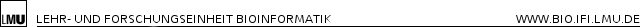# LFC

Next-generation-sequencing is the established method to quantify DNA/RNA by counting sequenced reads. Sampling noise of read counts can be controlled using Poisson based probabilistic models and inherent bias is assumed to cancel out when fold changes between two experimental conditions are computed. Here, we introduce a fundamentally different approach by directly modeling count ratios and reveal that bias also affects fold changes severely. Our model suggests a simple way to remove such bias and we show that corrected fold changes significantly outperform the standard count ratios. Our Bayesian method allows to utilize prior knowledge and to compute accurate normalization constants. Furthermore, credible intervals for fold changes can be computed, which is of interest especially for entities with few reads.Differential analysis of NGS data starts with the aligned reads of two conditions, here exemplified as RNA-seq reads from samples A and B aligned to an mRNA. Existing models take one specific route through the necessary steps defined in the main text: (I) For each sample, reads are aggregated and an appropriate probabilistic model is used to control noise and estimate the sample specific mRNA abundance. (II) These abundance estimates are then divided to give an estimate of the mRNA fold change. Our approach takes a different route by first computing local ratios for all read sequences and then aggregating them using an appropriate noise model for count ratios to estimate the total mRNA fold change. Using a basic noise model for the second step makes both routes equivalent. However, using extensions to it leads to more accurate fold change estimates by exploiting the fact that bias cancels out when taking the ratio of counts of individual sequences. Our implementation also allows to analyze replicate experiments: Either, all possible pairwise fold changes with associated credible intervals can be computed, or an average fold change by summing across all replicates from the same condition (in an appropriate way, see below).

###### Servicebereich
Informationen für